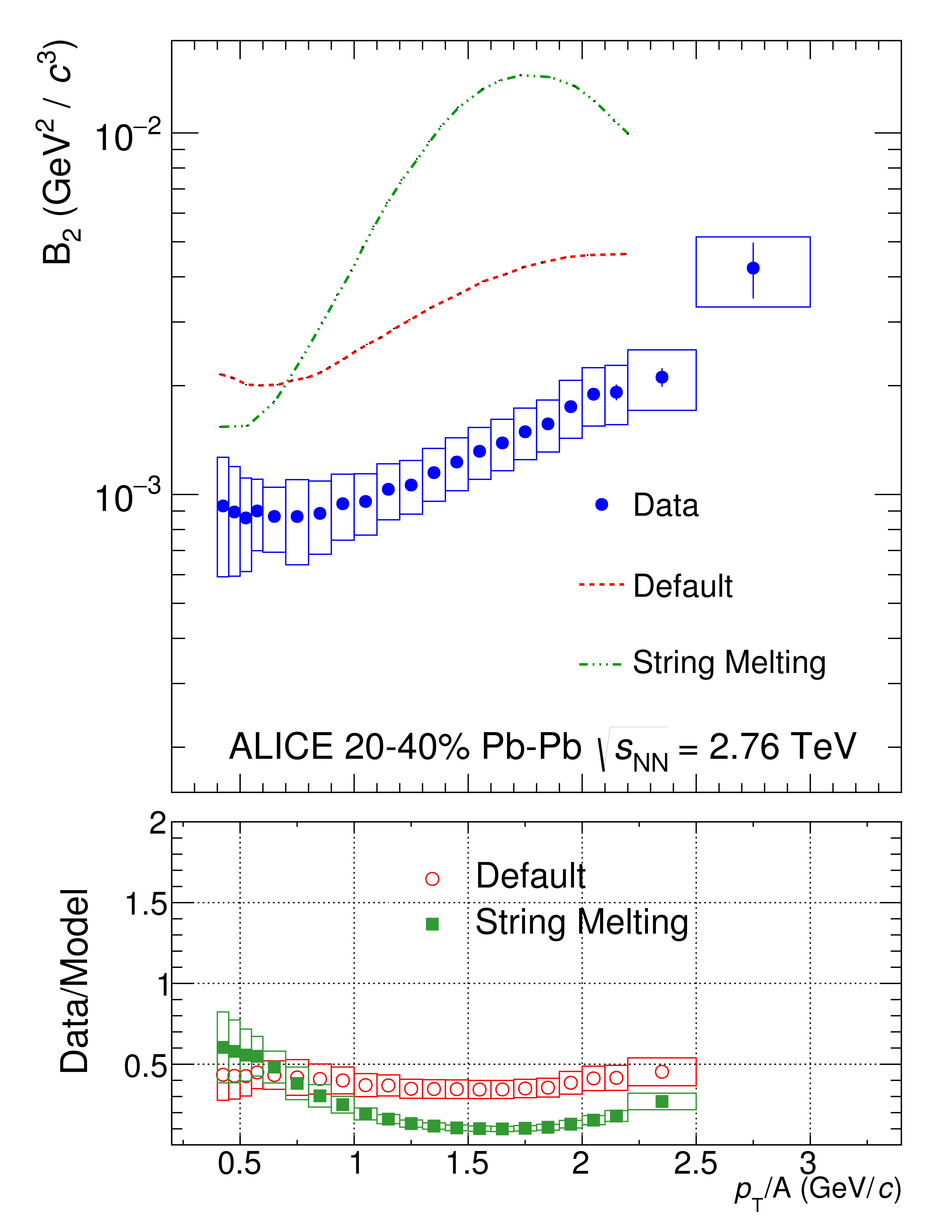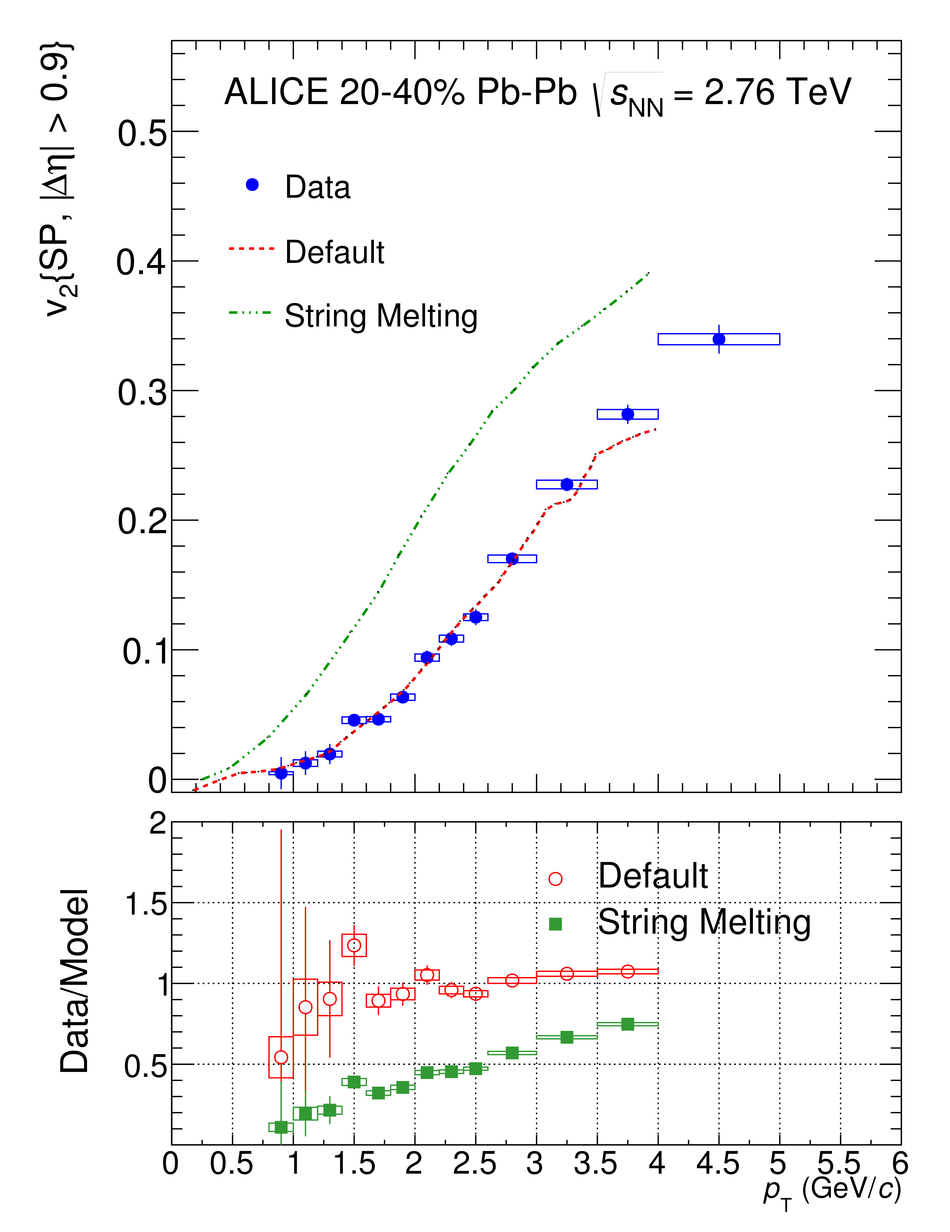# Measurement of deuteron spectra and elliptic flow in Pb-Pb collisions at $\mathbf{\sqrt{s_{\rm NN}}}$ = 2.76 TeV at the LHC

The transverse momentum ($p_{\rm T}$) spectra and elliptic flow coefficient ($v_2$) of deuterons and anti-deuterons at mid-rapidity ($|y|<~0.5$) are measured with the ALICE detector at the LHC in Pb-Pb collisions at $\sqrt{s_{\rm NN}} = 2.76$ TeV. The measurement of the $p_{\rm T}$ spectra of (anti-)deuterons is done up to 8 GeV/$c$ in 0-10% centrality class and up to 6 GeV/$c$ in 10-20% and 20-40% centrality classes. The $v_2$ is measured in the $0.8 <~p_{\rm T} <~5$ GeV/$c$ interval and in six different centrality intervals (0-5%, 5-10%, 10-20%, 20-30%, 30-40% and 40-50%) using the scalar product technique. Measured $\pi^{\pm}$, K$^{\pm}$ and p+$\overline{\mathrm{p}}$ transverse-momentum spectra and $v_2$ are used to predict the deuteron $p_{\rm T}$ spectra and $v_2$ within the Blast-Wave model. The predictions are able to reproduce the $v_2$ coefficient in the measured $p_{\rm T}$ range and the transverse-momentum spectra for $p_{\rm T}>1.8$ GeV/$c$ within the experimental uncertainties. The measurement of the coalescence parameter $B_2$ is performed, showing a $p_{\rm T}$ dependence in contrast with the simplest coalescence model, which fails to reproduce also the measured $v_2$ coefficient. In addition, the coalescence parameter $B_2$ and the elliptic flow coefficient in the 20-40% centrality interval are compared with the AMPT model which is able, in its version without string melting, to reproduce the measured $v_2$($p_{\rm T}$) and the $B_2$($p_{\rm T}$) trend.

Figures

## Figure 1

 The $m^2-m_{\mathrm{PDG}}^2$ distributions obtained using the TOF detector (left) and with the HMPID detector (right) in two different \pt\ intervals($4.4\leq\pt< 5$ \gmom\ and $5\leq\pt< 6$ \gmom) for positive tracks in the \mbox{0--10\%} centrality class. Here $m_{\mathrm{PDG}}$ is the nominal mass of deuteron asreported in~ Solid lines represent the total fit (signal plus background), dotted lines correspond to background and dashed lines to deuterons signal.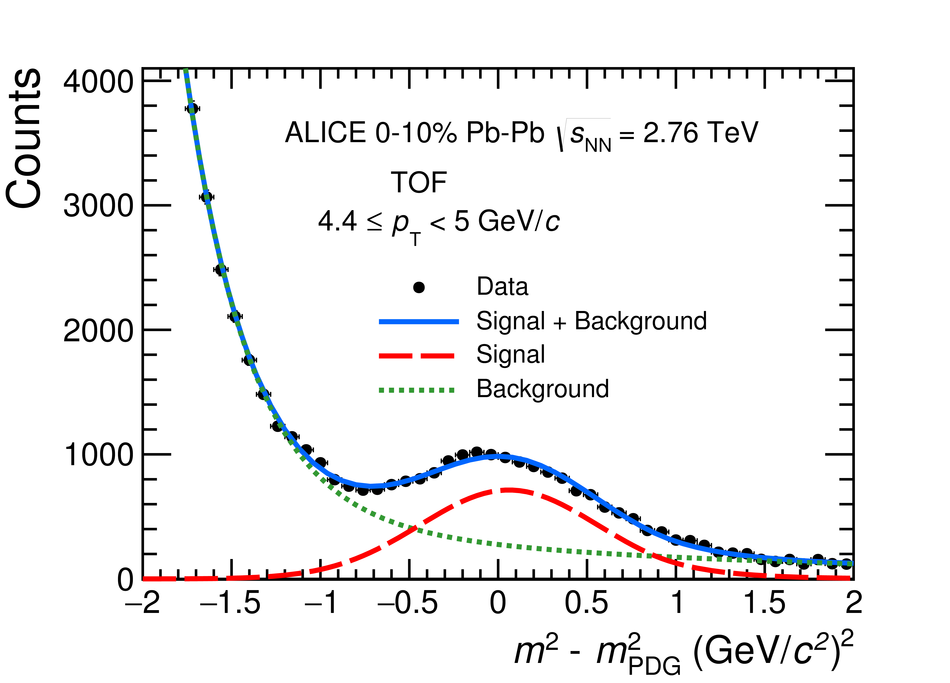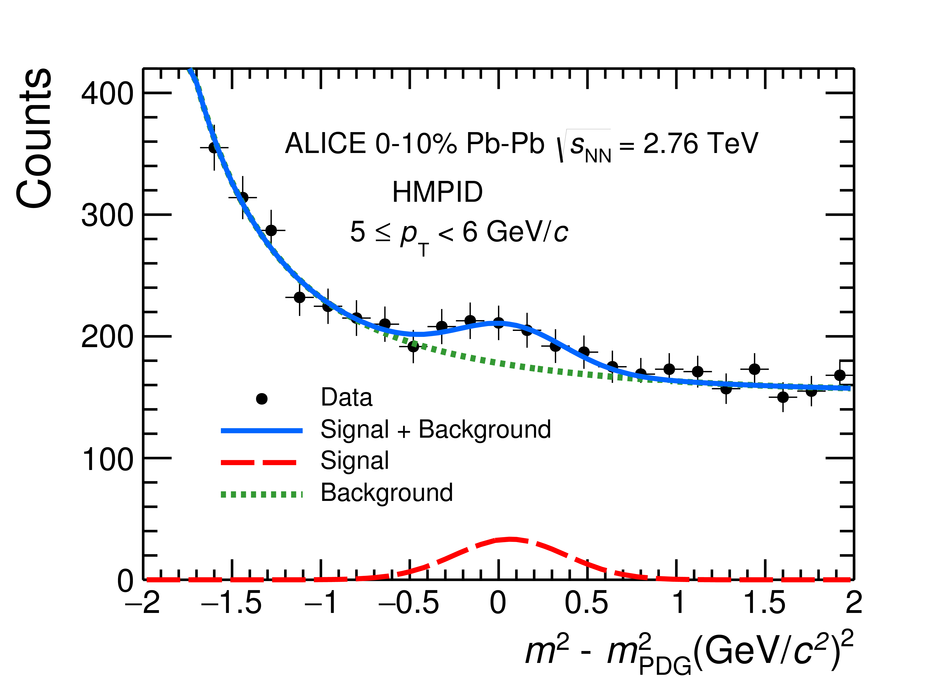## Figure 2

 Acceptance $\times$ efficiency (A $\times \epsilon$) as a function of transverse momentum for deuterons (filled markers) and anti--deuterons (open markers) in the most 10\%central \PbPb\ collisions at \s\ ~=~2.76 TeV for TPC-TOFand HMPID (multiplied by a scaling factor) analyses The TPC-TOF points account for tracking, matching efficiency and geometrical acceptance The dashed and solid curves represent the fits with the function presented in equation~\ref{eq:lineTOF} for deuterons and anti--deuterons respectively (see text for details) The HMPID points take into account tracking efficiency, geometrical acceptance, $\epsilon_\mathrm{{dist}}$ ( distance correction factor" as explained in the text) and PID efficiency. The lower value with respect to the TPC-TOF ismainly due to the limited geometrical acceptance of the HMPID detector (5\%).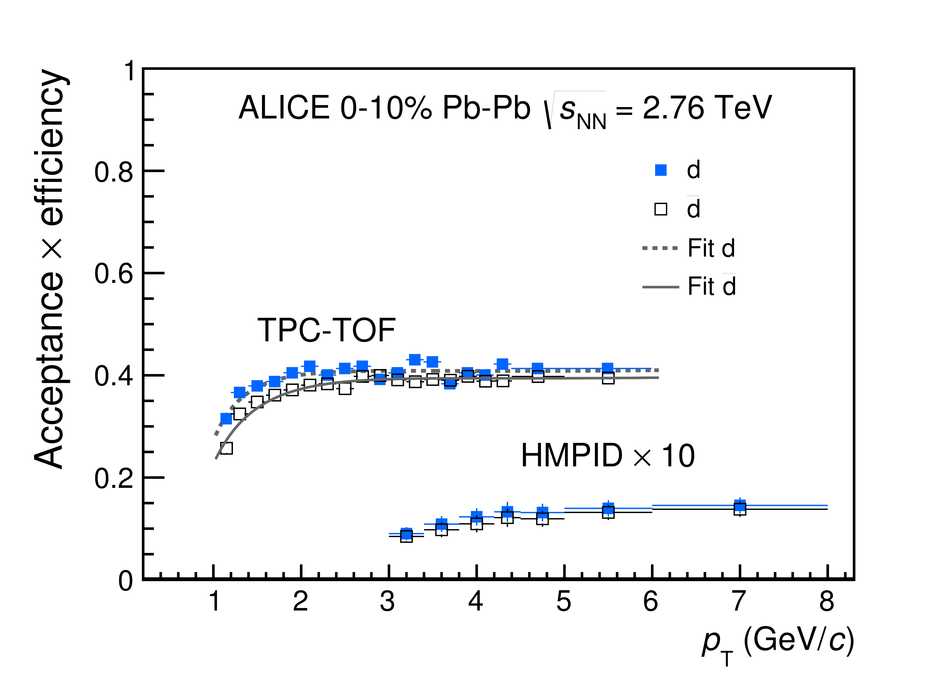## Figure 3

 In the upper panel the deuteron \pt\ spectra are shown for the three centrality intervals extended to high \pt\ with the TOF and HMPID analyses In the lower panels the ratios of anti-deuterons and deuterons are shown for the 0--10\%, 10--20\% and 20--40\% centrality intervals, from top to bottom The ratios are consistent with unity over the whole \pt\ range covered by the presented analyses.## Figure 4

 Left: Distribution of $\Delta \rm{M}$ for d+\dbar\ in the $2.2~\leq$~\pt~$< 2.4$~\gmom\and centrality interval 30-40\% fitted with a Gaussian with an exponential used to reproduce the signal andan exponential to reproduce the background Right: The \vtwo$^{\rm{Tot}}$ vs $\Delta \rm{M}$ for d+\dbar\ for 2.2~$\leq$~\pt~$<$~2.4~\gmom\in events with 30-40\% centrality. Points represent the measured $v_2^{\mathrm{Tot}}$,while the curve is the fit performed using equation~\ref{eq:v2tot}.## Figure 5

 Measured \vtwo\ as a function of \pt\ for \dbar\ (\pt~$<$~1.4~\gmom) and d+\dbar\(\pt~$\geq$~1.4~\gmom) for different centrality intervals in \mbox{\PbPb} collisionsat \s~=~2.76~TeV. Vertical bars represent statistical errors, while boxes are systematic uncertainties.## Figure 6

 \vtwo\ of $\pi^{\pm}$ (empty circles), p+$\overline{\rm p}$ (filled squares) and d+\dbar\ (filled circles) measured inthe 20--40\% centrality interval. A detailed description of each panel can be found in the text.## Figure 7

 Combined Blast-Wave fit to \pt\ and \vtwo(\pt) distributions usingequations \ref{eq:spectrum} and \ref{eq:v2}. The six upperpanels show the \pt\ spectra and the ratio between data and fit, while thesix panels in the bottom part shows the \vtwo(\pt) and the ratio betweendata and fit. In each panel, $\pi^\pm$ (empty circles), K$^\pm$ (diamonds),p+$\overline{\rm p}$ (filled squares) and d+\dbar\ (filled circles) are shown. For$\pi^\pm$, K$^\pm$ and p+$\overline{\rm p}$ the long dashedcurves represent the combined \pt\ and \vtwo\ Blast-Wave fit. Deuteron curves (dash dotted lines)are predictions from lighter particles Blast-Wave combined fit. Each column shows a different centralityintervals (0-10\% left, 10-20\% middle and 20-40\% right).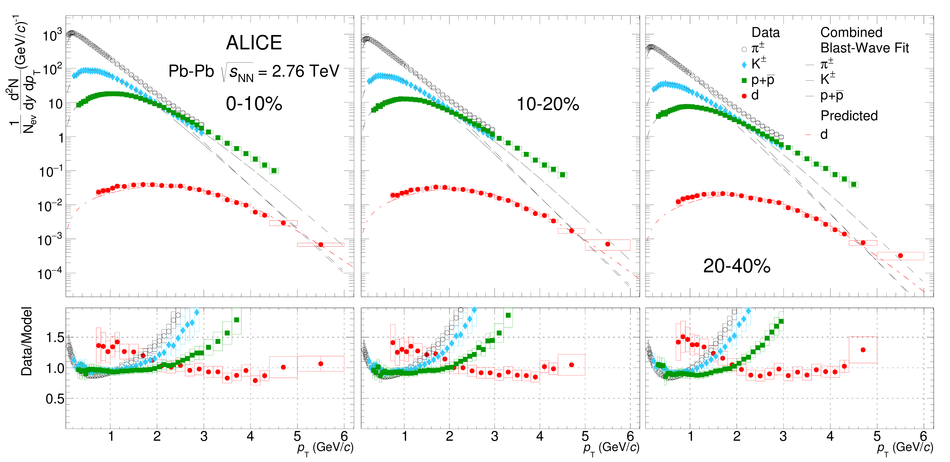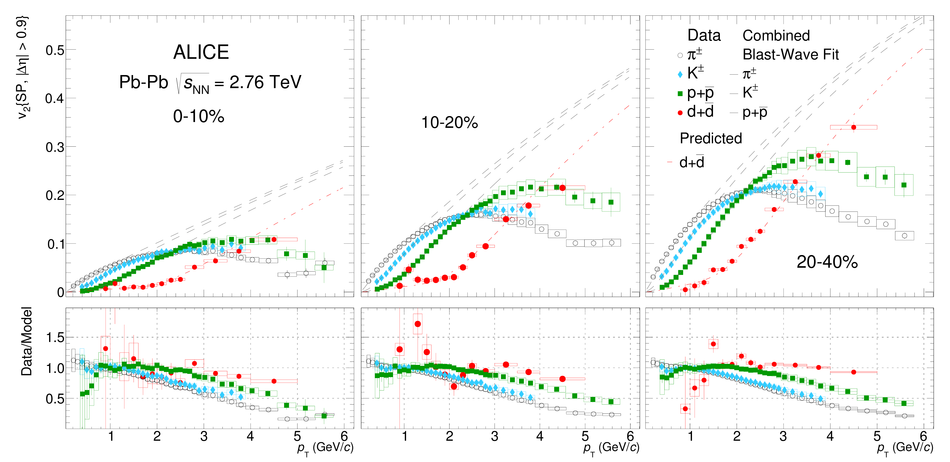## Figure 8

 Left: Coalescence parameter $B_2$ as a function of the transverse momentum per nucleon (\pt/A) fordifferent centrality classes. Right: Measured \vtwo\ of d+\dbar\ compared with the expectations from simplecoalescence (equation~\ref{eq:v2coal}) for different centrality intervals as indicated in the legend.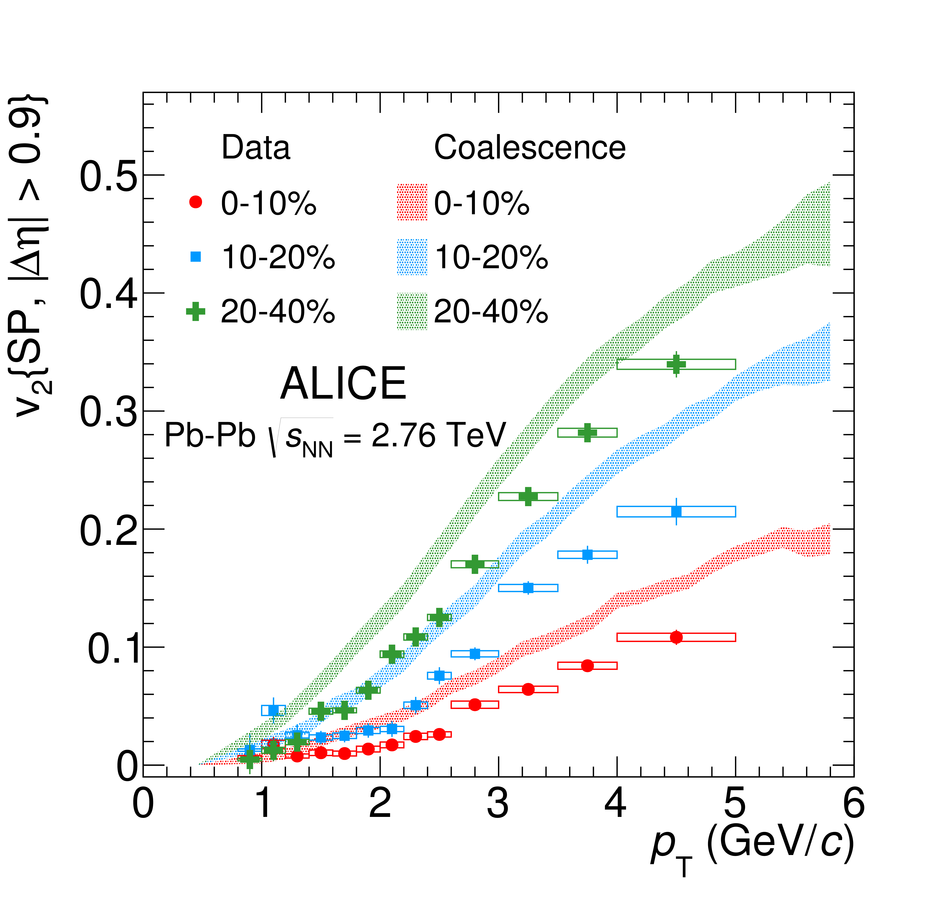## Figure 9

 Measured deuteron (filled dots) $B_2$ as a function of the transverse momentumper nucleon (\pt/A) (top left panel) and \vtwo(\pt) parameter (top right panel)compared to those produced with two versions of the AMPT model (dashed and dotted lines) In the bottom panels, the ratios between the measured data and the expectations fromthe two models are shown.